# Difference between Minkowski and Hamming distance

## Minkowski Distance

Minkowski Distance is the generalized form of Euclidean and Manhattan Distance.

The formula for Minkowski Distance is given as: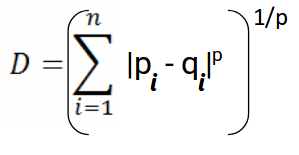Here, p represents the order of the norm. Let’s calculate the Minkowski Distance of the order 3:

# computing the minkowski distance
minkowski_distance = distance.minkowski(point_1, point_2, p=3)
print(‘Minkowski Distance b/w’, point_1, ‘and’, point_2, 'is: ', minkowski_distance)

view rawminkowski_distance.py hosted with by GitHub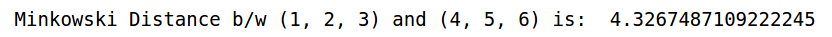The p parameter of the Minkowski Distance metric of SciPy represents the order of the norm. When the order§ is 1, it will represent Manhattan Distance and when the order in the above formula is 2, it will represent Euclidean Distance.

Let’s verify that in Python:

# minkowski and manhattan distance
minkowski_distance_order_1 = distance.minkowski(point_1, point_2, p=1)
print(‘Minkowski Distance of order 1:’,minkowski_distance_order_1, '\nManhattan Distance: ',manhattan_distance)

view rawminkowski_distance_vs_manhattan_distance.py hosted with by GitHub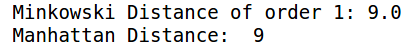Here, you can see that when the order is 1, both Minkowski and Manhattan Distance are the same. Let’s verify the Euclidean Distance as well:

# minkowski and euclidean distance
minkowski_distance_order_2 = distance.minkowski(point_1, point_2, p=2)
print(‘Minkowski Distance of order 2:’,minkowski_distance_order_2, '\nEuclidean Distance: ',euclidean_distance)

view rawminkowski_distance_vs_euclidean_distance.py hosted with by GitHub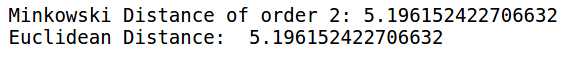When the order is 2, we can see that Minkowski and Euclidean distances are the same.

So far, we have covered the distance metrics that are used when we are dealing with continuous or numerical variables. But what if we have categorical variables? How can we decide the similarity between categorical variables? This is where we can make use of another distance metric called Hamming Distance.

## Hamming Distance

Hamming Distance measures the similarity between two strings of the same length. The Hamming Distance between two strings of the same length is the number of positions at which the corresponding characters are different.

Let’s understand the concept using an example. Let’s say we have two strings:

“euclidean” and “manhattan”

Since the length of these strings is equal, we can calculate the Hamming Distance. We will go character by character and match the strings. The first character of both the strings (e and m respectively) is different. Similarly, the second character of both the strings (u and a) is different. and so on.

Look carefully – seven characters are different whereas two characters (the last two characters) are similar: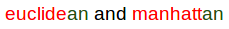Hence, the Hamming Distance here will be 7. Note that larger the Hamming Distance between two strings, more dissimilar will be those strings (and vice versa).

Let’s see how we can compute the Hamming Distance of two strings in Python. First, we’ll define two strings that we will be using:

# defining two strings
string_1 = ‘euclidean’
string_2 = ‘manhattan’

view rawdata_hamming.py hosted with by GitHub

These are the two strings “euclidean” and “manhattan” which we have seen in the example as well. Let’s now calculate the Hamming distance between these two strings:

# computing the hamming distance
hamming_distance = distance.hamming(list(string_1), list(string_2))*len(string_1)
print(‘Hamming Distance b/w’, string_1, ‘and’, string_2, 'is: ', hamming_distance)

view rawhamming_distance.py hosted with by GitHub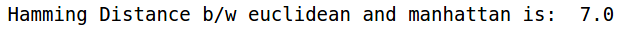As we saw in the example above, the Hamming Distance between “euclidean” and “manhattan” is 7. We also saw that Hamming Distance only works when we have strings of the same length.

Let’s see what happens when we have strings of different lengths:

# strings of different shapes
new_string_1 = ‘data’
new_string_2 = ‘science’
len(new_string_1), len(new_string_2)

view rawdata_hamming_2.py hosted with by GitHub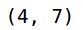You can see that the lengths of both the strings are different. Let’s see what will happen when we try to calculate the Hamming Distance between these two strings:

# computing the hamming distance
hamming_distance = distance.hamming(list(new_string_1), list(new_string_2))

view rawhamming_different_shape.py hosted with by GitHub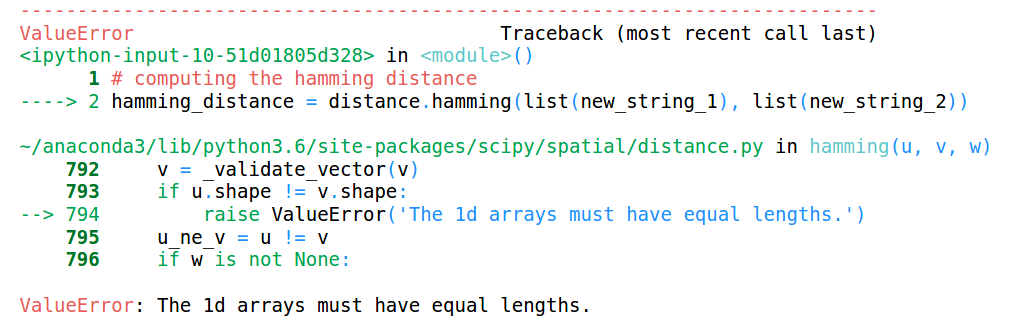This throws an error saying that the lengths of the arrays must be the same. Hence, Hamming distance only works when we have strings or arrays of the same length.